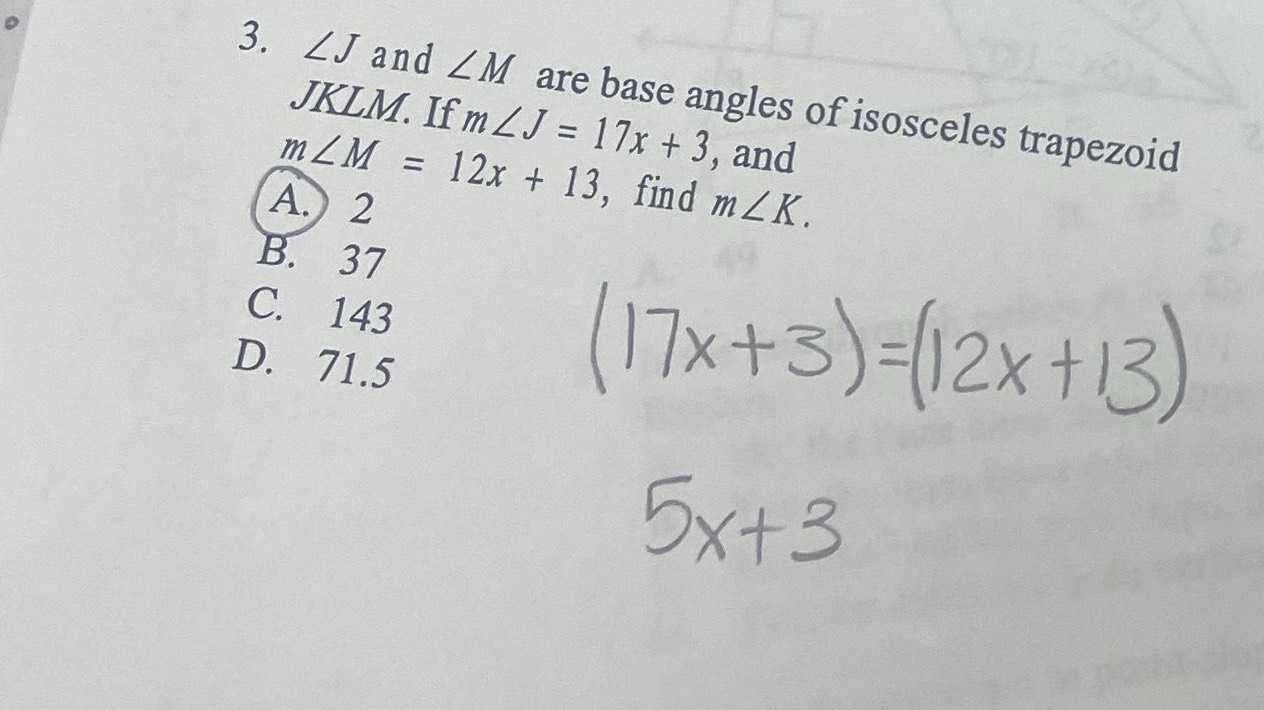### ¿Todavía tienes preguntas de matemáticas?

Pregunte a nuestros tutores expertos
Algebra
Pregunta3. $$\angle J$$ and $$\angle M$$ are base angles of isosceles trapezoid JKLM. If $$m \angle J = 17 x + 3$$ , and

$$m \angle M = 12 x + 13$$ , find $$m \angle K$$ .

A. $$2$$

B. $$37$$

C. $$143$$

D. $$71.5$$